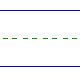Name: ______________________________________________ Date: _____________

Instructions: Find the sum.

 6 + 0 =8 + 2 =6 + 4 =0 + 9 =2 + 5 =5 + 4 =3 + 2 =9 + 0 =4 + 1 =6 + 1 =1 + 9 =5 + 1 =2 + 3 =4 + 4 =1 + 4 =3 + 6 =7 + 1 =7 + 3 =